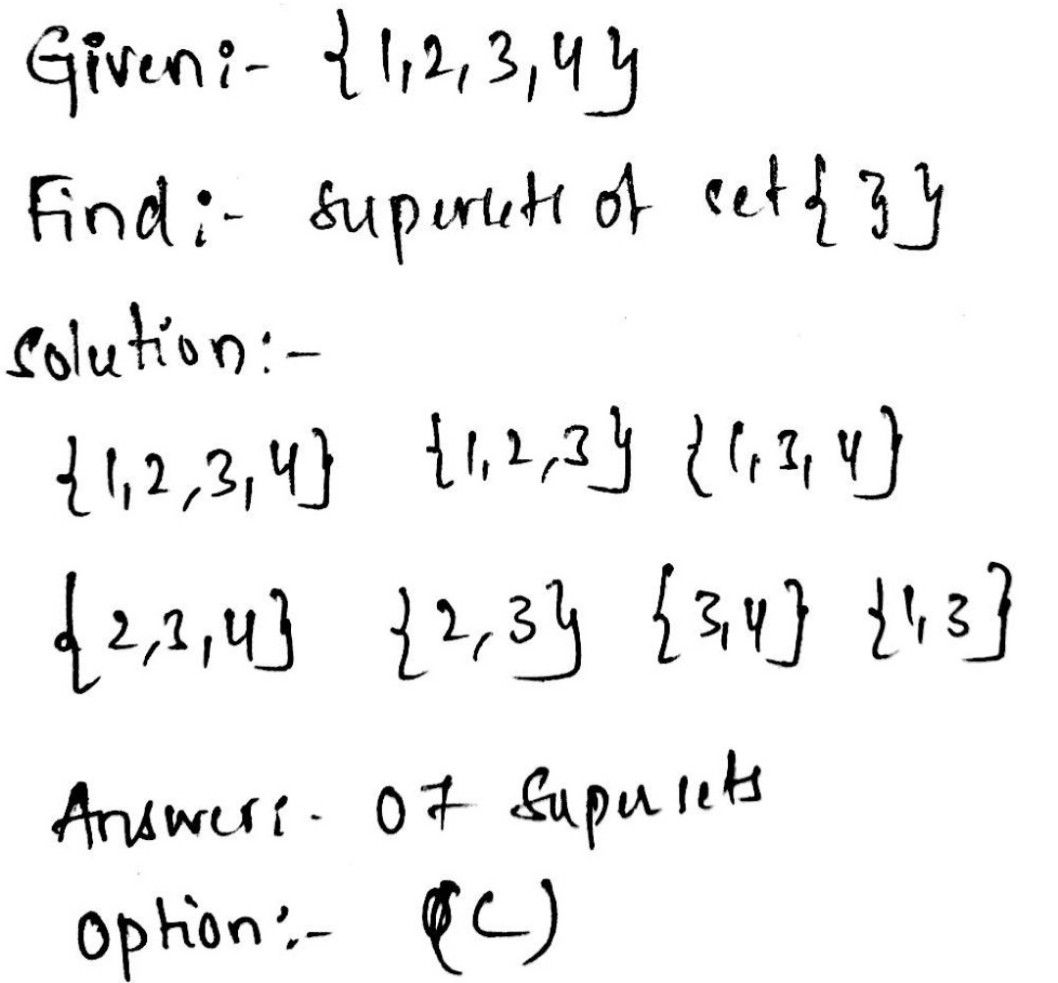Symbol
Problem$2.$ Consider the proper subsets of $\left(1,2,3,4\right)$ How many of these proper subsets are superset of the set $\left(3\right)$ ? $\left($ (a) $\right)$ $5$ $\left($ (b) $0\right)$ $6$ $\left($ (c) $7$ $\left($ (d) $\right)$ $8$